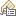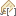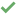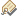# Calculate Max Elevation Figures (Topographic Production)

## サマリー

Calculates the maximum elevation figures (MEF) for each polygon cell or quadrangle in a polygon layer. You use these values as labels for the MEF feature layer.

To derive the highest obstruction value, the tool uses terrain data from a digital elevation model (DEM) and vertical obstruction data from a point feature class. You can calculate MEF values for a Joint Operations Graphic (JOG), an Operational Navigation Chart (ONC), Tactical Pilotage Chart (TPC) grid cells, NATO STANAG-3591 Edition 6, or any other polygon grid features.

## 使用法

• This tool will modify existing features by updating selected fields.

• You can label the maximum elevation figures in the MEF feature layer. Complete the following steps to apply the label expression:

1. Run the Calculate Max Elevation Figures tool on the MEF feature layer.
2. Right-click the MEF feature layer in the Contents pane and click Labeling Properties.

The Label Class pane appears.

3. Click the Label expression tab.
4. For language, choose VBScript.
5. Load the expression below into the Expression window. Change all [MEF_VALUE] occurrences to the name of the mef_field.
``````Function FindLabel ( [MEF_VALUE] )
Dim l, h, s, f, z, j, len2, lenfin
s = [MEF_VALUE]
len2 = len(s)
If len2 < 4 then
l = mid(s, 1, 1)
z = "<FNT name='Arial' size='30'>" & "0" & "</FNT>"
j = "<SUP><FNT name='Arial' size='30'>" & l & "</FNT></SUP>"
Findlabel = z & j
ElseIf len2 = 4 then
l = mid(s, 1, 1)
h = mid(s, 2, 1)
z = "<FNT name='Arial' size='30'>" & l & "</FNT>"
j = "<SUP><FNT name='Arial' size='30'>" & h & "</FNT></SUP>"
Findlabel = Z & j
Else
l = mid(s, 1, 2)
h = mid(s, 3, 1)
z = "<FNT name='Arial' size='30'>" & l & "</FNT>"
j = "<SUP><FNT name='Arial' size='30'>" & h & "</FNT></SUP>"
Findlabel = Z & j
End If
End Function``````
6. Click Verifyto validate the label expression.
7. Click Apply.
8. Click the Labeling tab for the feature layer.
9. In the Layer group, click Enable Labeling.

## パラメーター

 ラベル 説明 データ タイプ Target Max Elevation Figures Features The input polygon features representing the quadrangle or cell that will be updated with MEF values. Feature Layer Input Terrain The input terrain that will be used to determine elevation values in an MEF feature cell. If a point feature layer is used, elevation values are obtained from the field defined in the Terrain Elevation Field parameter. Raster Layer; Mosaic Layer; Layer Vertical Obstruction Features The layers that will be used to identify the highest human-made structure within a cell. This is a value table defining Features, Elevation Field, and Elevation Unit. Value Table Max Elevation Figures Field The existing field in the Target Max Elevation Figures Features layer where the maximum elevation figure value will be stored. Field Max Vertical Obstruction Field The field in the Target Max Elevation Figures Features layer where the maximum vertical obstruction value will be stored. Field Max Terrain Field The field in the Target Max Elevation Figures Features layer where the maximum elevation values from the terrain layer will be stored. Field Specification Specifies the specification that will be used to calculate maximum elevation figures.JOG-MIL-J-89100—The Joint Operations Graphic specification will be used.ONC-MIL-O-89102—The Operational Navigation Chart specification will be used.TPC-MIL-T-89101—The Tactical Pilotage Chart will be used.STANAG-3591-ED6—The NATO Standard Agreement will be used. String Terrain Elevation Field (オプション) A field in the Input Terrain value that represents the elevation values for each feature. If a point feature layer is used for the Input Terrain parameter, this parameter is required. This parameter is inactive if a raster or mosaic layer is used as input for the Input Terrain parameter. Field Vertical Obstruction Allowance (オプション) A vertical allowance value that will be added to each calculated MEF value. The value accounts for nonrepresented natural or manufactured features. The default is 150 feet. Linear Unit Vertical Obstruction Accuracy (オプション) The accuracy of the vertical obstruction feature layer within a specified number of units. The default is 20 meters. Linear Unit Terrain Accuracy(オプション) The accuracy of the terrain layer within a specified number of units. The default is 20 meters. Linear Unit

### 派生した出力

 ラベル 説明 データ タイプ Updated Max Elevation Figures Features The Target Max Elevation Figures Features parameter value with updated MEF values. Feature Layer

`arcpy.topographic.CalculateMEF(target_mef_features, in_terrain, obstruction_features, mef_field, max_vo_field, max_terrain_field, specification, {terrain_elev_field}, {vo_allowance}, {vo_accuracy}, {terrain_accuracy})`
 名前 説明 データ タイプ target_mef_features The input polygon features representing the quadrangle or cell that will be updated with MEF values. Feature Layer in_terrain[in_terrain,...] The input terrain that will be used to determine elevation values within an MEF feature cell. If a point feature layer is used, elevation values are obtained from the field defined in the terrain_elev_field parameter. Raster Layer; Mosaic Layer; Layer obstruction_features[obstruction_features,...] The layers that will be used to identify the highest human-made structure within a cell. This is a value table defining Features, Elevation Field, and Elevation Unit. Value Table mef_field The existing field in the target_mef_features layer where the maximum elevation figure value will be stored. Field max_vo_field The field in the target_mef_features layer where the maximum vertical obstruction value will be stored. Field max_terrain_field The field in the target_mef_features layer where the maximum elevation values from the terrain layer will be stored. Field specification Specifies the specification that will be used to calculate maximum elevation figures.JOG_MIL_J_89100—The Joint Operations Graphic specification will be used.ONC_MIL_O_89102—The Operational Navigation Chart specification will be used.TPC_MIL_T_89101—The Tactical Pilotage Chart will be used.STANAG_3591_ED6—The NATO Standard Agreement will be used. String terrain_elev_field(オプション) A field in the in_terrain value that represents the elevation values for each feature. If a point feature layer is used for the in_terrain parameter, this parameter is required. This parameter is disabled if a raster or mosaic layer is used as input for the in_terrain parameter. Field vo_allowance(オプション) A vertical allowance value that will be added to each calculated MEF value. The value accounts for nonrepresented natural or manufactured features. The default is 150 feet. Linear Unit vo_accuracy(オプション) The accuracy of the vertical obstruction feature layer within a specified number of units. The default is 20 meters. Linear Unit terrain_accuracy(オプション) The accuracy of the terrain layer within a specified number of units. The default is 20 meters. Linear Unit

### 派生した出力

 名前 説明 データ タイプ updated_mef_features The target_mef_features parameter value with updated MEF values. Feature Layer

### コードのサンプル

CalculateMaxElevationFigures example (stand-alone script)

The following code sample demonstrates how to use the CalculateMaxElevationFigures function in Python.

``````# Name: CalculateMaxElevationFigures_sample.py
# Description: Calculates maximum elevation figures values for JOG data.

# Import System Modules
import arcpy

# Check Out Extensions
arcpy.CheckOutExtension('Foundation')

# Setting the environment
arcpy.env.workspace = r'C:\Data\JOG.gdb\BM'
arcpy.env.overwriteOutput = True

# Make Feature Layer for Obstruction Features
arcpy.MakeFeatureLayer_management(r'VerticalObstructionPnt', 'VO_Layer')

# Setting Local Variables
target_mef_features = r'MaximumElevationSrf'
in_terrain = r'C:\Data\w120n34.dt2;C:\Data\w119n34.dt2'
obstruction_features = r'VO_Layer ZVH Meters'
mef_field = 'MEF_VALUE'
max_vo_field = 'MAX_ELEVATION'
max_terrain_field = 'MAX_TERRAIN'
specification = 'JOG_MIL_J_89100'
terrain_elev_field = ''
vo_allowance = '150 Feet'
vo_accuracy = '20 Meters'
terrain_accuracy = '20 Meters'

# Use the Calculate Max Elevation Figures GP tool to calculate maximum elevation figures values for JOG data
arcpy.topographic.CalculateMEF(target_mef_features, in_terrain, obstruction_features, mef_field, max_vo_field, max_terrain_field, specification, terrain_elev_field, vo_allowance, vo_accuracy, terrain_accuracy)

# Check In Extensions
arcpy.CheckInExtension('Foundation')``````

## ライセンス情報

• Basic: No
• Standard: 次のものが必要 Production Mapping